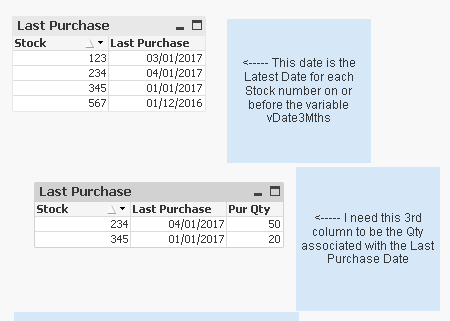# QlikView App Development

Discussion Board for collaboration related to QlikView App Development.Partner

## Dynamic Set Expression inside a Set Expression

Hi All,

I think I have an easy question, however, I must be having a mental block, as the answer escapes me. Hopefully, someone can assist.

I have a Fact table with a mixture of Stock Purchase information and Stock Sales information.

My ultimate goal is to show a list of Stock Items (in a straight table) that were Purchased more than 3 months ago, and have only sold less than 50% of the Purchase Qty.

Conditions:

1. Only show Stock Items that have sold less than 50% of Purchase Qty

2. Only show Stock Items that are associated with the most recent Purchase, before 3 month ago (eg, if I have 3 Purchases dated 01-Mar-2017, 15-Mar-2017, 10-Apr-2017, and today = 21-Jun-2017, I only want Stock Items on the 15-Mar-2017)

3. The main dimension will be Stock Item, and each Stock Item must satisfy Conditions 1. and 2.

4. A variable is required to determine when 3 months ago is.

5. Purchase Qty is calculated on or before the date variable.

6. Sales Qty is calculated on or after the date variable.

Columns to be shown will be:-

Stock Item                         Dimension

Item Description              Dimension

Purchase Qty                    Expression

Sold Qty                             Expression

Last Purchase Date        Expression

Last Sold Date                 Expression

The first issue surrounds calculating the Purchase Qty and the Sold Qty, as these are required to restrict the Stock Items shown.

I believe I will need an If condition on every Expression to achieve the correct Stock List.

Something like:

If((Sold Qty / Purchased Qty) < 0.5, Some Expression, 0)

Both Sold Qty and Purchased Qty are tricky, as both are based on the Last Purchase Date and each Stock Item (each Row) will have a different Date that the Purchase was done. It could be any date before the variable date (vDate3Mths).

For Sales - Expression would be like Sum({<Date = {All dates after the Last Purchase Date}>} Qty * isSLine)

This is what I thought may work Sum({<Date = {"=\$(=Max({<isPLine = {1}, Date = {'<=\$(vDate3Mths)'}>} Date))"}>} Qty * isSLine)

This part is what I use to calculate the latest Purchase date before the variable date

Max({<isPLine = {1}, Date = {'<=\$(vDate3Mths)'}>} Date)

But when used inside another Set Expression, it is not dynamic for each Stock Item. I think I need to somehow use Aggr() function, however, not sure of the syntax.

The exact same issue is with the Purchased Qty

I have attached a simple example .qvw

1 Solution

Accepted SolutionsMVP

## Re: Dynamic Set Expression inside a Set Expression

May be this

Sum({<isPLine = {1}, Date = {'<=\$(vDate3Mths)'}>}Aggr(If(\$(vTest50) and Date = Max(TOTAL <Stock>{<isPLine = {1}, Date = {'<=\$(vDate3Mths)'}>} Date), Max({<isPLine = {1}, Date = {'<=\$(vDate3Mths)'}>} Qty), 0), Stock, Date))7 RepliesMVP

## Re: Dynamic Set Expression inside a Set Expression

How you are calculating 3 months before here?

I mean with respect to today's date?

If yes, provide data accordingly.Partner

## Re: Dynamic Set Expression inside a Set Expression

Hi Manish,

Thanks for the response.

In my real QlikView document the expression for vDate3Mths:

=Date(AddMonths(Max({<isSLine = {1}>} Date), -3))

Which calculates 3 months before the latest Sales Invoice (Which may not be today() or the date of reload. Done this as there is Budget data for the entire year.

In my example qvw, which you have, I use a similar Expression:

=Date(Max(Date) - 2)

The concept is the same. Changing the example qvw variable to =Date(Max({<isSLine = {1}>} Date) - 2) is essentially the same and should demonstrate the same result.

I can't provide data due to client confidentiality, and size of document.MVP

## Re: Dynamic Set Expression inside a Set Expression

May be this

Sum({<isPLine = {1}, Date = {'<=\$(vDate3Mths)'}>}Aggr(If(\$(vTest50) and Date = Max(TOTAL <Stock>{<isPLine = {1}, Date = {'<=\$(vDate3Mths)'}>} Date), Max({<isPLine = {1}, Date = {'<=\$(vDate3Mths)'}>} Qty), 0), Stock, Date))Partner

## Re: Dynamic Set Expression inside a Set Expression

Hi Sunny,

Thanks for the reply. That expression doesn't satisfy the conditions.

Stock 123 & 567 should not be in the table as more than 50% of the Purchase Qty has been sold.

I think vTest50 is the problem, as I know it contains the incorrect expression for Purchased Qty and Sold QtyMVP

## Re: Dynamic Set Expression inside a Set Expression

May be try this

Sum({<isPLine = {1}, Date = {'<=\$(vDate3Mths)'}>}

Aggr(

If(

Sum(TOTAL <Stock> {<Date = {'>=\$(vDate3Mths)'}>} Qty * isSLine) / Max(TOTAL <Stock>{<isPLine = {1}, Date = {'<=\$(vDate3Mths)'}>} Qty) < 0.5 and

Date = Max(TOTAL <Stock>{<isPLine = {1}, Date = {'<=\$(vDate3Mths)'}>} Date) and

Sum(TOTAL <Stock> {<Date = {'>=\$(vDate3Mths)'}>} Qty * isSLine) > 0,

Max({<isPLine = {1}, Date = {'<=\$(vDate3Mths)'}>} Qty), 0)

, Stock, Date))Partner

## Re: Dynamic Set Expression inside a Set Expression

Hi Sunny,

I decided to use a version of this response. Thank you.

vTest50 = Sum(Aggr(If(Date >= Max(TOTAL <Stock>{<isPLine = {1}, Date = {'<=\$(vDate3Mths)'}>} Date), Sum(Qty * isSLine), 0), Stock, Date)) / Sum({<isPLine = {1}, Date = {'<=\$(vDate3Mths)'}>} Aggr(If(Date = Max(TOTAL <Stock>{<isPLine = {1}, Date = {'<=\$(vDate3Mths)'}>} Date), Max({<isPLine = {1}, Date = {'<=\$(vDate3Mths)'}>} Qty), 0), Stock, Date)) < 0.5

Last Purchase = If(\$(vTest50), Max({<isPLine = {1}, Date = {'<=\$(vDate3Mths)'}>} Date), Null())

Last Sale = If(\$(vTest50), Max({<isSLine = {1}>} Date), Null())

Purchase Qty = If(\$(vTest50), Sum({<isPLine = {1}, Date = {'<=\$(vDate3Mths)'}>}Aggr(If(Date = Max(TOTAL <Stock>{<isPLine = {1}, Date = {'<=\$(vDate3Mths)'}>} Date), Max({<isPLine = {1}, Date = {'<=\$(vDate3Mths)'}>} Qty), 0), Stock, Date)), Null())

Sold Qty = If(\$(vTest50), Sum(Aggr(If(Date >= Max(TOTAL <Stock>{<isPLine = {1}, Date = {'<=\$(vDate3Mths)'}>} Date), Sum(Qty * isSLine), 0), Stock, Date)), Null())MVP

## Re: Dynamic Set Expression inside a Set Expression

So everything working then? If it is, I would suggest you to close this thread by marking correct response

Qlik Community Tip: Marking Replies as Correct or Helpful

Best,

Sunny